The AUTOREG Procedure

## Example 8.6 Estimation of ARCH(2) Process

Stock returns show a tendency for small changes to be followed by small changes while large changes are followed by large changes. The plot of daily price changes of IBM common stock (Box and Jenkins; 1976, p. 527) is shown in Output 8.6.1. The time series look serially uncorrelated, but the plot makes us skeptical of their independence.

With the following DATA step, the stock (capital) returns are computed from the closing prices. To forecast the conditional variance, an additional 46 observations with missing values are generated.

```title 'IBM Stock Returns (daily)';
title2 '29jun1959 - 30jun1960';

data ibm;
infile datalines eof=last;
input x @@;
r = dif( log( x ) );
time = _n_-1;
output;
return;
last:
do i = 1 to 46;
r = .;
time + 1;
output;
end;
return;
datalines;

... more lines ...

```
``` proc sgplot data=ibm;
series y=r x=time/lineattrs=(color=blue);
refline 0/ axis = y LINEATTRS = (pattern=ShortDash);
run;
```

Output 8.6.1 IBM Stock Returns: Daily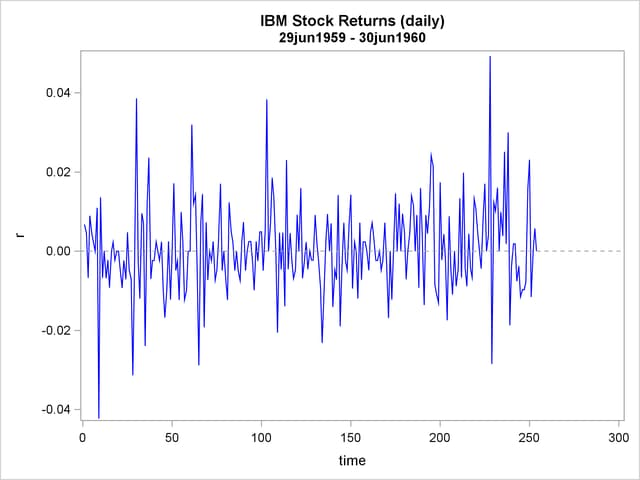The simple ARCH(2) model is estimated using the AUTOREG procedure. The MODEL statement option GARCH=(Q=2) specifies the ARCH(2) model. The OUTPUT statement with the CEV= option produces the conditional variances V. The conditional variance and its forecast are calculated using parameter estimates: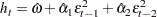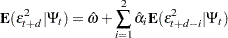where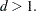This model can be estimated as follows:

```proc autoreg data=ibm maxit=50;
model r = / noint garch=(q=2);
output out=a cev=v;
run;
```

The parameter estimates for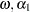, and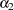are 0.00011, 0.04136, and 0.06976, respectively. The normality test indicates that the conditional normal distribution may not fully explain the leptokurtosis in the stock returns (Bollerslev; 1987).

The ARCH model estimates are shown in Output 8.6.2, and conditional variances are also shown in Output 8.6.3. The code that generates Output 8.6.3 is shown below.

```data b; set a;
length type \$ 8.;
if r ^= . then do;
type = 'ESTIMATE'; output; end;
else do;
type = 'FORECAST'; output; end;
run;
proc sgplot data=b;
series x=time y=v/group=type;
refline 254/ axis = x LINEATTRS = (pattern=ShortDash);
run;
```

Output 8.6.2 ARCH(2) Estimation Results
 IBM Stock Returns (daily) 29jun1959 - 30jun1960

The AUTOREG Procedure

Dependent Variable r

Ordinary Least Squares Estimates
SSE 0.03214307 DFE 254
MSE 0.0001265 Root MSE 0.01125
SBC -1558.802 AIC -1558.802
MAE 0.00814086 AICC -1558.802
MAPE 100.378566 HQC -1558.802
Durbin-Watson 2.1377 Regress R-Square 0.0000
Total R-Square 0.0000

 Algorithm converged.

GARCH Estimates
SSE 0.03214307 Observations 254
MSE 0.0001265 Uncond Var 0.00012632
Log Likelihood 781.017441 Total R-Square 0.0000
SBC -1545.4229 AIC -1556.0349
MAE 0.00805675 AICC -1555.9389
MAPE 100 HQC -1551.7658
Normality Test 105.8587
Pr > ChiSq <.0001

Parameter Estimates
Variable DF Estimate Standard Error t Value Approx
Pr > |t|
ARCH0 1 0.000112 7.6059E-6 14.76 <.0001
ARCH1 1 0.0414 0.0514 0.81 0.4208
ARCH2 1 0.0698 0.0434 1.61 0.1082

Output 8.6.3 Conditional Variance for IBM Stock Prices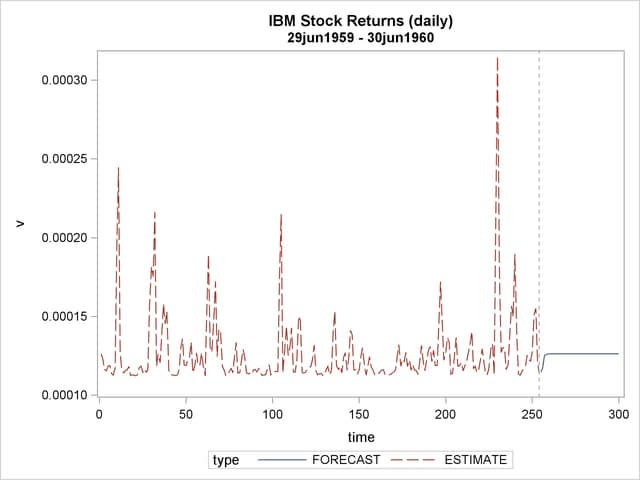Previous Page | Next Page | Top of Page